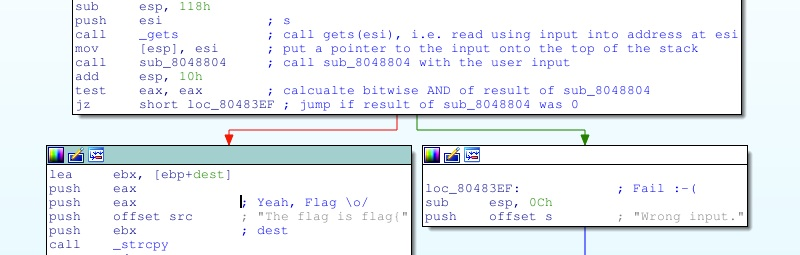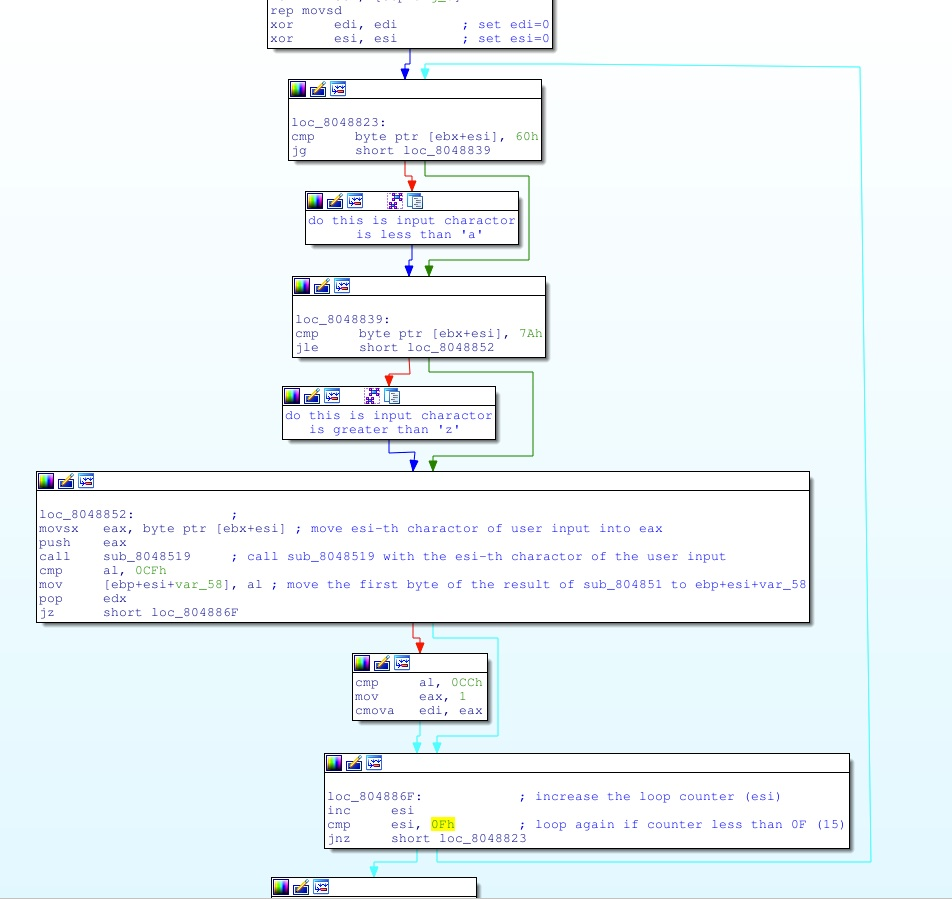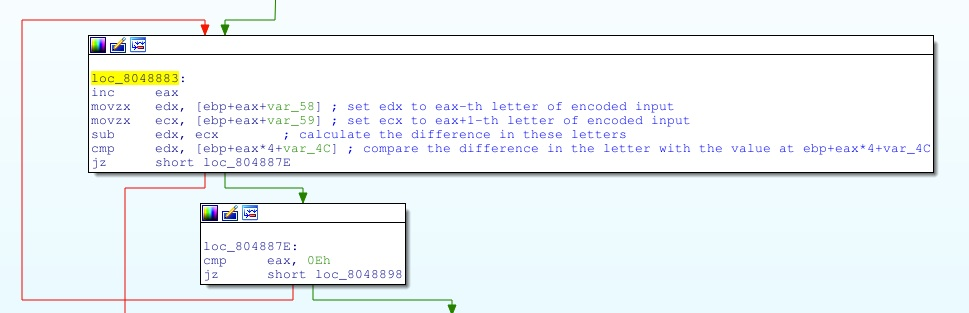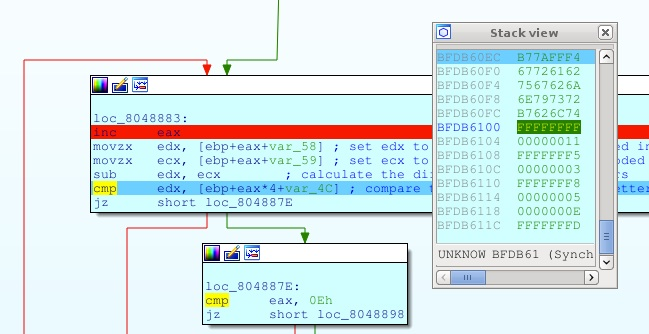Jul 12, 2015 • Abstract

The challenges description provides the file ‘reversemeplz’, which can be found here, and ‘file’ identifies it as a 32-bit linux executable:

So we fire up a 32-bit linux VM and open it into the free demo version of IDA pro. Now we go through the code using ‘:’ to add comments to each line and ‘N’ to rename any functions and memory location.

The main function (with our comments) is as follows:From this we see that the executable reads a line of user input using ‘gets’, and then calls the ‘sub_8048804’ function on it. If the result of this function is 0, the executable prints ‘Wrong input’ but if it’s none zero then the program adds “flag{“ and “}” on either end. I.e., We need to find an input that makes ‘sub_8048804’ return true.

Looking at ‘sub_8048804’ we see it starts with a loop:Before the start of the loop esi is set to 0, and at the end it’s incremented and tested against 0Fh (15), so the program loops round 15 times.

The input will be sitting just above the ebx pointer, so ‘byte ptr [ebx+esi]’ will be the esi-th character of the input. The loop checks that this character of the input is between 60h and 7Ah (i.e., it’s a lower case letter), so far most of the flags we saw in PoliCTF were lower case, so we ignore what the program does if the character is not a lower case letter.

The body of the loop then calls ‘sub_8048519’ on each character of the input in turn and puts the first byte of the result onto the stack at ‘ebp+var_58’.

‘sub_8048519’ is a very long an complex function so we simply run the program with all lower case letters as input in the IDA debugger and see what we get back. We are in luck ‘sub_8048519’ is not a stateful function and we quickly spot that it in fact calculates ROT13!

After calculating the ROT13 of the input the next part of ‘sub_8048519’ goes into another loop:Recall that the result of the ‘sub_8048519’ was put at ‘ebp+var_58’, so this code puts each consecutive pair of letters into edx and ecx, it then calculates the difference between these letters and compares it with the value at ‘[ebp+eax*4+var_4C]’. To find out what these values are we run the program in the IDA debugger and take a look for ourselves:We see that the difference between the 1st and the 2nd ROT13 encoded character must be FFFFFFFF (-1) and the difference between the 2nd and the 3th must be 11, the 3rd and the fourth FFFFFFF5 (-11), and so on. Guessing that the flag is English words, we find that ‘onetwotheflagyo’ matches this, and running the executable confirms that this is the flag: